Level

Lots of examples using Level can be found in the discussion of Level Specification. Below I explain some of the subtle details.

In the next cell we get  a list of all subexpressions at level 2 in expr.  Notice x1 and  x7  each  appear twice in expr and twice in the result returned by Level.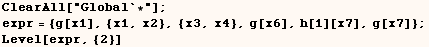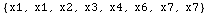It seems Level gives a result such that the elements are sorted according to
their position in the original expression.  This is demonstrated in the next
cell where a sorted list of positions is used to get the same result we got
above and the positions are in canonical order.  Notice each list of
positions has length 2 and this is what we expect since we asked for all
expressions at level 2.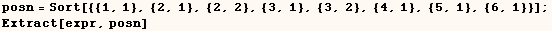Heads Option

By default Level uses the setting (Heads→False).  In the next cell we
use (Heads→True) and get the heads at level 2.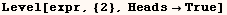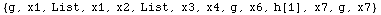Using the third argument in Level

Finally Level can be given a third argument that can be applied to the list
returned.  To demonstrate this feature consider the next cell which gives a
list of all subexpressions at level 3 and deeper.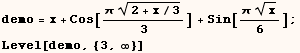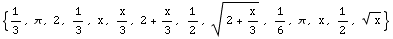In the next cell Level returns the sum of the elements we got from the
previous cell.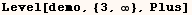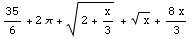Created by Mathematica  (May 16, 2004)

Back to Ted’s Tricks index page